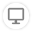100
Views
4
Solved
###### [MathUtils] Wrong calculations
QuestionForge component by Justin James

Hello there,

I'm using this component in a project where i need to use some trigonometry functions.

While debugging some calculations, i found out that, at least, the Sin() function is not calculating the values as it should. These are some examples:If i calculate the sin of 38,722252 on my TI-84, it returns 0,62554... In the Sin() functions of this component, the value returned is highlighted in the image above.

Another example is:Again, in my TI-84 the sin of 55,562 is 0,8247... and in the Sin() function it returns a negative number, that considering the trigonometric circle, that's not even possible. My TI-84 is in degrees by the way, not in radians.

Thank you,

Rafael ValenteLeonardo Fernandes
MVP
Solution

Hi Rafael.

The trigonometric functions are working in radians. To take a sine of an angle measured in degrees, first you need to multiply your angle by 2*pi/360, which is roughly 0.017453.

In your example, to calculate sin(38.722252 deg) you would need to multiply the angle, and only then take the sine function.

sin(0.017453 * 38.722252) = sin(0.675819) = 0.62554

sin(0.017453 * 55.562) = sin(0.969724) = 0.82473Rafael Valente

Leonardo Fernandes wrote:

Hi Rafael.

The trigonometric functions are working in radians. To take a sine of an angle measured in degrees, first you need to multiply your angle by 2*pi/360, which is roughly 0.017453.

In your example, to calculate sin(38.722252 deg) you would need to multiply the angle, and only then take the sine function.

sin(0.017453 * 38.722252) = sin(0.675819) = 0.62554

sin(0.017453 * 55.562) = sin(0.969724) = 0.82473

Hello Leonardo,

In the other discussion i opened, @JustinJames replied that the calculations are made in degrees.

But after confirming your calculations, you are absolutely right!

Thank you a lot!

Best regards,

Rafael ValenteJustin James
MVP

Rafael -

This is nothing that can be done in the Extension. All this does is call the .NET and Java functions of the right way and pass the answer back. If the answer is not right, it is either because of some of the known issues in OutSystems around decimals due to casting under the hood (the Trunc() function has some issues...) or it is .NET/Java that is not calculating things as you expect. Suggest you try a test in a console application on the same platform you are working on (.NET or Java) to validate where the issues are coming from.

J.JaLeonardo Fernandes
MVP
Solution

Hi Rafael.

The trigonometric functions are working in radians. To take a sine of an angle measured in degrees, first you need to multiply your angle by 2*pi/360, which is roughly 0.017453.

In your example, to calculate sin(38.722252 deg) you would need to multiply the angle, and only then take the sine function.

sin(0.017453 * 38.722252) = sin(0.675819) = 0.62554

sin(0.017453 * 55.562) = sin(0.969724) = 0.82473Rafael Valente

Leonardo Fernandes wrote:

Hi Rafael.

The trigonometric functions are working in radians. To take a sine of an angle measured in degrees, first you need to multiply your angle by 2*pi/360, which is roughly 0.017453.

In your example, to calculate sin(38.722252 deg) you would need to multiply the angle, and only then take the sine function.

sin(0.017453 * 38.722252) = sin(0.675819) = 0.62554

sin(0.017453 * 55.562) = sin(0.969724) = 0.82473

Hello Leonardo,

In the other discussion i opened, @JustinJames replied that the calculations are made in degrees.

But after confirming your calculations, you are absolutely right!

Thank you a lot!

Best regards,

Rafael ValenteLeonardo Fernandes